Next: Conditional Density Estimation Up: Learning: Conditional vs. Joint Previous: Squandered Resources: The Shoe

# Conditional Densities: A Bayesian Framework

In Bayesian inference, the probability density function of a vector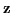is typically estimated from a training set of such vectors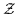as shown in Equation 5.1 .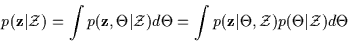(5.1)

By integrating over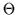, we are essentially integrating over all the pdf models possible. This involves varying the families of pdfs and all their parameters. However, often, this is impossible and instead a family is selected and only its parametrizationis varied. Eachis a parametrization of the pdf ofand is weighted by its likelihood given the training set. However, computing the integral 5.1 is not always straightforward and Bayesian inference is approximated via maximum a posteriori (MAP) or maximum likelihood (ML) estimation as in Equation 5.2. The EM algorithm is frequently utilized to perform these maximizations.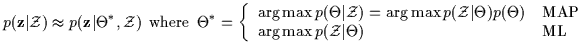(5.2)Next: Conditional Density Estimation Up: Learning: Conditional vs. Joint Previous: Squandered Resources: The Shoe
Tony Jebara
1999-09-15